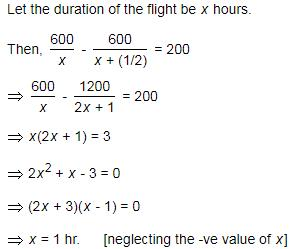Courses

# Test: Speed, Time And Distance- 2

## 10 Questions MCQ Test Quantitative Aptitude for SSC CGL | Test: Speed, Time And Distance- 2

Description
This mock test of Test: Speed, Time And Distance- 2 for Quant helps you for every Quant entrance exam. This contains 10 Multiple Choice Questions for Quant Test: Speed, Time And Distance- 2 (mcq) to study with solutions a complete question bank. The solved questions answers in this Test: Speed, Time And Distance- 2 quiz give you a good mix of easy questions and tough questions. Quant students definitely take this Test: Speed, Time And Distance- 2 exercise for a better result in the exam. You can find other Test: Speed, Time And Distance- 2 extra questions, long questions & short questions for Quant on EduRev as well by searching above.
QUESTION: 1

Solution:
QUESTION: 2

Solution:
QUESTION: 3

### It takes eight hours for a 600 km journey, if 120 km is done by train and the rest by car. It takes 20 minutes more, if 200 km is done by train and the rest by car. What is the ratio of the speed of the train to that of the car?

Solution:
QUESTION: 4
If a person walks at 14 km/hr instead of 10 km/hr, he would have walked 20 km more. What is the actual distance travelled by him?
Solution:
QUESTION: 5

In a flight of 600 km, an aircraft was slowed down due to bad weather. Its average speed for the trip was reduced by 200 km/hr and the time of flight increased by 30 minutes. What is the duration of the flight ?

Solution:option "D"

QUESTION: 6

A Man travelled a distance of 61 km in 9 hours. He travelled partly on foot at 4 km/hr and partly on bicycle at 9 km/hr. What is the distance travelled on foot?

Solution:

Let the time in which he travelled on foot = x hour
Time for travelling on bicycle = (9 - x) hr

Distance = Speed * Time, and Total distance = 61 km
So,
4x + 9(9-x) = 61
=> 5x = 20
=> x = 4

So distance traveled on foot = 4(4) = 16 km

QUESTION: 7
A man rides his bicycle 10 km at an average speed of 12 km/hr and again travels 12 km at an average speed of 10 km/hr. What is his average speed for the entire trip approximately?
Solution:
QUESTION: 8
The ratio between the speeds of two trains is 7 : 8. If the second train runs 400 km in 4 hours, What is the the speed of the first train?
Solution:
QUESTION: 9
It takes eight hours for a 600 km journey, if 120 km is done by train and the rest by car. It takes 20 minutes more, if 200 km is done by train and the rest by car. What is the ratio of the speed of the train to that of the car?
Solution:
QUESTION: 10
Walking 6/7th of his usual speed, a man is 12 minutes too late. What is the usual time taken by him to cover that distance?
Solution: